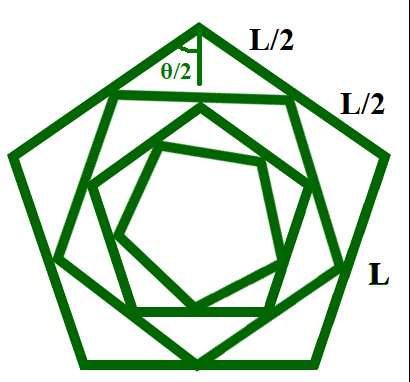Skip to content
Related Articles
Find the length of Kth N-sided polygon formed by given operations
• Last Updated : 10 May, 2021

Given an integer L, representing the side length of an N-sided regular polygon and integer K, the task is to find the side length of the Kth N-sided regular polygon formed inside the (K – 1)th regular polygon by connecting midpoints of the sides of the (K – 1)th polygon.

Examples:

Input: N = 3, L = 6, K = 2
Output: 3

Input: N = 5, L = 21, K = 7
Output: 5.88796

Approach: The given problem can be solved based on the following observations:• Suppose,  \theta represents the interior angle of the N-sided polygon which is the same for all the polygons formed inside i.e.,• The length of the side of the first polygon formed inside by connecting midpoints of the sides can be calculated using the formula as.
• The length of the side of the Kth polygon formed inside the (K – 1)th polygon and connecting midpoints of the sides of the (K – 1)th polygon isFollow the steps below to solve the problem:

• Find the interior angle of the N-sided regular polygon and store it in a variable say angle in radians.
• Print the side length after calculating the side length of Kth N sided regular polygon by the above-discussed formula.

Below is the implementation of the above approach:

## C++

 // C++ program for the above approach #include using namespace std;#define PI 3.14159265 // Function to calculate the interior// angle of a N-sided regular polygondouble findInteriorAngle(int n){    return (n - 2) * PI / n;} // Function to find the K-th polygon// formed inside the (K - 1)th polygondouble calculateSideLength(double L,                           int N, int K){    // Stores the interior angle    double angle = findInteriorAngle(N);     // Stores the side length of    // K-th regular polygon    double length = L * pow(sin(angle / 2),                            (K - 1));     // Return the length    return length;} // Driver Codeint main(){    double N = 5, L = 21, K = 7;    cout << calculateSideLength(L, N, K);     return 0;}

## Java

 // Java program for the above approachimport java.util.*; class GFG{     static final double PI = 3.14159265; // Function to calculate the interior// angle of a N-sided regular polygonstatic double findInteriorAngle(int n){    return ((n - 2) * PI) / n;} // Function to find the K-th polygon// formed inside the (K - 1)th polygonstatic double calculateSideLength(double L,                                  int N, int K){         // Stores the interior angle    double angle = findInteriorAngle(N);     // Stores the side length of    // K-th regular polygon    double length = L * Math.pow(Math.sin(angle / 2),                                             (K - 1));     // Return the length    return length;} // Driver Codepublic static void main(String[] args){    double L = 21;    int N = 5, K = 7;         System.out.print(calculateSideLength(L, N, K));}} // This code is contributed by 29AjayKumar

## Python3

 # Python3 program for the above approachimport mathPI = 3.14159265 # Function to calculate the interior# angle of a N-sided regular polygondef findInteriorAngle(n):     return (n - 2) * PI / n # Function to find the K-th polygon# formed inside the (K - 1)th polygondef calculateSideLength(L,                        N, K):     # Stores the interior angle    angle = findInteriorAngle(N)     # Stores the side length of    # K-th regular polygon    length = L * pow(math.sin(angle / 2),                     (K - 1))     # Return the length    return length  # Driver Codeif __name__ == "__main__":     N = 5    L = 21    K = 7    print(calculateSideLength(L, N, K))     # This code is contributed by ukasp.

## C#

 // C# program for the above approachusing System; class GFG{     static readonly double PI = 3.14159265; // Function to calculate the interior// angle of a N-sided regular polygonstatic double findInteriorAngle(int n){    return ((n - 2) * PI) / n;} // Function to find the K-th polygon// formed inside the (K - 1)th polygonstatic double calculateSideLength(double L,                                  int N, int K){         // Stores the interior angle    double angle = findInteriorAngle(N);     // Stores the side length of    // K-th regular polygon    double length = L * Math.Pow(Math.Sin(angle / 2),                                             (K - 1));     // Return the length    return length;} // Driver Codepublic static void Main(String[] args){    double L = 21;    int N = 5, K = 7;         Console.Write(calculateSideLength(L, N, K));}} // This code is contributed by 29AjayKumar

## Javascript

 
Output:
5.88796

Time Complexity: O(log K)
Auxiliary Space: O(1)

Attention reader! Don’t stop learning now. Get hold of all the important DSA concepts with the DSA Self Paced Course at a student-friendly price and become industry ready.  To complete your preparation from learning a language to DS Algo and many more,  please refer Complete Interview Preparation Course.

In case you wish to attend live classes with industry experts, please refer Geeks Classes Live

My Personal Notes arrow_drop_up